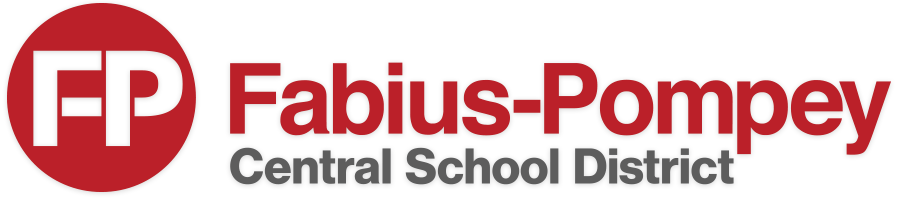Mr. Gilbert's Math 8 Curriculum
The following list of topics shows the topics we will cover in Math 8 this year, separated by unit, along with a general time frame.
KEY:

Red - Unit has been completed
Green - Unit is in progress
Black - Unit is still to come

Unit 1 - Angles and Shapes (September)

• Angle pairs (supplementary, complementary, vertical, adjacent angles)*
• Parallel lines cut by a transversal
• Angle sum and exterior angles of triangles
• Similar triangles
• Constructing triangles*
• Surface Area*
• Volume
• Cross-Sections*

Unit 2 - Equations with one variable (October)

• Solving two-step equations (review)
• Combining Like Terms
• Equations with the distributive property
• Equations with combining like terms
• Equations with variables on both sides
• Multi-step equations
• Equations with fractions

Unit 3 - Linear Functions (October - December)
• Definition of a function
• Graphs of Lines (y = mx and y = mx + b)
• Slope and y-intercept
• Multiple representations of functions (Graphs, tables, equations, verbal descriptions)
• Linear vs non-linear functions
• Writing linear functions
• Describing graphs
• Drawing graphs

Unit 4 - Statistics (December-January)
• Constructing and describing scatter plots
• Best fit lines
• Interpret slope and y-intercept of best fit lines
• Two-way tables
Unit 5 - Equations with two variables (January)
• Solving graphically
• Solving with substitution
• Solving by elimination
• Solving by "inspection"
• Word problems

Unit 6 - Transformations (February - March)
• Translations
• Reflections
• Rotations
• Dilations
• Proving congruence
• Proving similarity

Unit 7 - Exponents (March)
• Negative and zero exponents
• Laws of Exponents (multiplying, dividing, power to a power)
• Scientific notation
• Operations with scientific notation
• Comparing with scientific notation

Unit 8 - State Test Review (April)
• Topics TBD

Unit 9 - Real Number System (May - June)
• Irrational numbers
• Decimal expansions
• Graphing on a number line
• Perfect squares and cubes
• Simple quadratic and cubic equations
• Pythagorean Theorem

DepartmentsAddress: 1211 Mill Street | Fabius, NY 13063
Phone: (315)683-5301